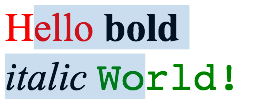Our DNA is written in Swift
Jump

# Color Math

For my Rich Text Editing component I wanted selections to look like the Apple originals. So I made a screenshot of some editing action and inspected it in Photoshop to find out how they are drawn.Visually there are two options: either the selection boxes are drawn behind the text not affecting it, or they are layered on top of the text.  Because the selection also changes the color values of the black text it must be the latter. It’s on top.

In this article I’ll try to figure out the math so that I can calculate the original blue and alpha value used. We will see that it is orders of magnitude easier to do if we know the result of blending this color over white and black than two arbitrary ones.

Assuming that a normal blend mode is used the math to compose two pixels and their alpha is according to Wikipedia.So we basically diminish the pixel color by the inverse of the alpha and add the new color of the blended pixel. Ok, that’s not too difficult.

Using the color dropper tool we find these values to be the the result of the blending:

Color + Black = Rb: 0 (0.000), Gb: 17 (0.067), Bb: 34 (0.133)
Color + White = Rw: 203 (0.796), Gw: 221 (0.867), Bw: 238 (0.933)

### Calculating Original Color

I turned to twitter for help and Andy Durdin from Edinburgh responded with the appropriate equations while standing and waiting for a bus.

Ro = Red of Original Color
Rw = Red Value from blending Ro with White
Rb = Red Value from blending Ro with Black
A = Alpha

Changing the formulas to look like the on quote above:

Rw = (1 – A) * 1 + A * Ro
Rb =  (1 – A) * 0 + A * Ro

Now my algebra is quite weak, but with Andy’s help I figured out

Rb = A * Ro
Rw = 1 – A + Rb

and then add A to and subtract Rw from both sides.

A = 1 + Rb – Rw
Ro = Rb / A

So lets substitute the Red values and see what we get as a result:

A = 1 + 0 – 0.796 = 0.204
Ro = 0 / 0.2 = 0
Go = 0.067 / 0.2 = 0.338
Bo = 0.133 / 0.2 = 0.652

Lo and behold, the color we get matches exactly!Being curious I asked Andy if this solving technique would work for any two arbitrary colors as well. Somehow I suspected that having black in there might make the whole way simpler because the multiplication with 0 reliminates a big chunk of one of the two equations. The answer is yes. If alpha is involved we always have two unknowns to solve for. You always require the same number of simultaneous equations as you have unknowns.

### Bonus: Arbitrary Colors

Andy sat down and flexed his iron math muscles a bit more. 🙂

A solid color C0 is blended with alpha A over two other colors C1, C2. The blend with C1 produces color C3, and the blend with C2 produces color C4.

Considering only the red channels (r0 = red channel of c0), we have:

R3 = A * R0 + (1 – A) * R1
R4 = A * R0 + (1 – A) * R2

If we only know C1, C2, C3, C4, we can solve for C0 and a. Again, considering just the red channels, a solution is:

s = (R2 – R1) / (R4 – R2)
R0 = (R2 * s – R1) / (s – 1)
A = (R4 – R2) / (R0 – R2)

s is used just to make the equation for r0 more readable.

Andy’s conclusion: “This is a solution, and it works, but I’m not very happy with it. With some colors it is extremely sensitive to the rounding errors in the colour values.”

So, yes, it’s possible, but not very feasible to try to get the original color and alpha when it’s blended with two arbitrary colors. So generally we’ll stick with white and black if we want to find out what original colors Apple is using for certain UI elements.